International
Tables for
Crystallography
Volume D
Physical properties of crystals
Edited by A. Authier

International Tables for Crystallography (2006). Vol. D, ch. 1.5, p. 146

## Section 1.5.10. Transformation from Gaussian to SI units

A. S. Borovik-Romanova and H. Grimmerb*

aP. L. Kapitza Institute for Physical Problems, Russian Academy of Sciences, Kosygin Street 2, 119334 Moscow, Russia, and bLabor für Neutronenstreuung, ETH Zurich, and Paul Scherrer Institute, CH-5234 Villigen PSI, Switzerland
Correspondence e-mail:  hans.grimmer@psi.ch

### 1.5.10. Transformation from Gaussian to SI units

| top | pdf |

Numerical values of magnetic quantities are given in Gaussian units in this chapter. For each quantity that appears in a table or figure, Table 1.5.10.1gives the corresponding Gaussian unit and its value expressed in SI units. More details on the transformation between Gaussian and SI units are given e.g. in the Appendix of Jackson (1999).

 Table 1.5.10.1| top | pdf | Conversion of Gaussian to SI units
SymbolQuantityGaussian unit and its SI equivalent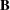Magnetic induction 1 gauss (G) = 10−4 tesla (T)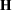Magnetic field 1 oersted (Oe) = 103/(4π) A m−1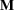Magnetization (= magnetic moment per unit volume) 1 emu cm−3 = 103 A m−1Linear magnetoelectric tensor (rationalized units) 1 (dimensionless units) = 4π × 10−8/3 s m−1Piezomagnetic tensor 1 Oe−1 = 4π × 10−3 m A−1 = 4π × 10−3 T Pa−1Magnetic volume susceptibility 1 (dimensionless units) = 4π (dimensionless units)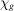Magnetic mass susceptibility 1 cm3 g−1 = 4π × 10−6 m3 g−1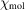Magnetic molar susceptibility 1 cm3 mol−1 = 4π × 10−6 m3 mol−1

### References

Jackson, J. D. (1999). Classical electrodynamics, 3rd ed. New York: Wiley.